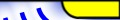Index    Chapter 1    Chapter 2    Chapter 3    Conclusion    Appendix    Matlab Code    Audio DemoCDMA Results

By Eric Lawrey, Copyright 1997 - 2001

## 3.1 Simulated Model

The forward link of the CDMA system modelled uses orthogonal Walsh codes to separate the users. Each user is randomly allocated a Walsh code to spread the data to be transmitted.

The transmitted signals from all the users are combined together, then passed through a radio channel model. This allows for clipping of the signal, adding multipath interference, and adding white gaussian noise to the signal.

The receiver uses the same Walsh code that was used by the transmitter to demodulate the signal and recover the data. After the received signal has been despread using the Walsh code, it is sub-sampled back down to the original data rate. This is done by using an integrate-and-dump filter, followed by a comparator to decide whether the data was a 1 or a 0.

The received data is then compared with the original data transmitted to calculate the bit error rate (BER).

The RMS amplitude error is also worked out. The signal level after it has been demodulated and filtered is compared with the expected amplitude of the signal based on the transmitted data. The RMS amplitude error directly relates to the bit error rate, so is a useful measurement to make.

Figure 33 shows the model used for the simulations of the CDMA forward link.Figure 33 Model used for the CDMA forward link.

### 3.1.2 Reverse Path

The reverse link of the CDMA link was simulated in a very similar way to the forward link except that orthogonal Walsh codes are not used. As shown earlier it is extremely difficult to use orthogonal codes effectively in a reverse link from the mobiles to the base station, because of the difficulty in synchronizing the system accurately enough. Because of this simply long pseudo random codes were used instead of the Walsh codes.

## 3.2 Simulation Results

### 3.2.1 BER verses the number of users in a cell

The reverse links of a CDMA system, from the mobiles to the base station, use non-orthogonal codes, which are pseudo random noise codes (PN codes). This leads to the signals from each user interfering with each other. The signals transmitted by each user are uncorrelated with each other as each user uses a unique pseudo random sequence code, resulting in the signal appearing a noise to other users.Figure 34 BER verse the number of users in a cell, for the reverse link in a CDMA system.

The BER for the reverse link of a CDMA system, increase as more users use the same cell. Figure 34 shows the BER expected base on the number of users in a cell. This result, is for an isolated cell with no interference from neighbouring cells, no multipath  effects, and no channel noise. Any of these effects would worsen the BER. From Figure 34 it can be seen that the BER becomes significantly large if the number of users is greater then 8 users. This represents only 12.5% of the total user capacity of 64 users. The maximum number of users in the cell can be increased by using, advanced forward error correction, voice activity detection, and cell sectorization.

For computer transfer applications such as wireless local area networks, the data load is generally in bursts. This allows a reduction in the duty cycle of each user which is similar to voice activity detection. This can lead to a vast increase in the number of users possible as the interference from each user is reduced. However, it is at the cost of total data throughput of each user.

It is clear from the result obtained from Figure 34 that the inter-user interference in the reverse link is the weak point in the CDMA system. It is this interference that limits the cell capacity to approximately 8-12 users.

### 3.2.2 Multipath Immunity

CDMA is inherently tolerant to multipath delay spread signals as any signal that is delayed by more than one chip time becomes uncorrelated to the PN code used to decode the signal. This results in the multipath simply appearing as noise. This noise leads to an increase in the amount of interference seen by each user subjected to the multipath and thus increases the received BER.

Figure 35 shows the effect of delay spread on the reverse link of a CDMA system. It can be seen that the BER is essentially flat for delay spreads of greater than one chip time (0.8 msec), which is to be expected as the reflected signal becomes uncorrelated.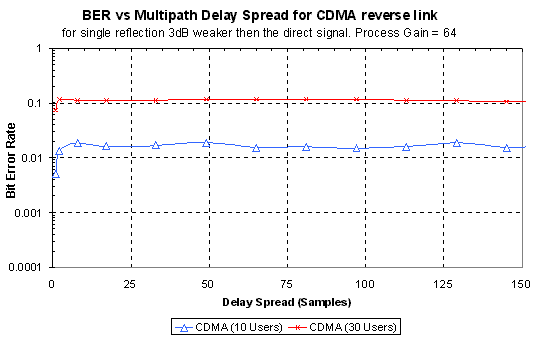Figure 35 Effect of multipath delay spread on the reverse link of a CDMA system.

The multipath delay spread leads to an increase in the equivalent number of users in the cell, as it increases the amount of interference seen by the receiver.

Figure 36 shows a how the multipath power leads to an increase in the effective number of users in the cell. This simulation was performed using a fixed number of users in the CDMA link. A multipath signal of 10 samples in delay (to ensure that it is uncorrelated) was then added. It was found that as the amplitude of the reflected signal was increased, so did the bit error rate (BER). This BER was compared with Figure 34 to find out the equivalent number of users that result in the same BER.

The addition of multipath to the signal increases the total interference in the cell. The level of this increase is proportional to the number of users in the cell and the multipath signal strength. Figure 36 also shows the predicted result based on the increase interference power.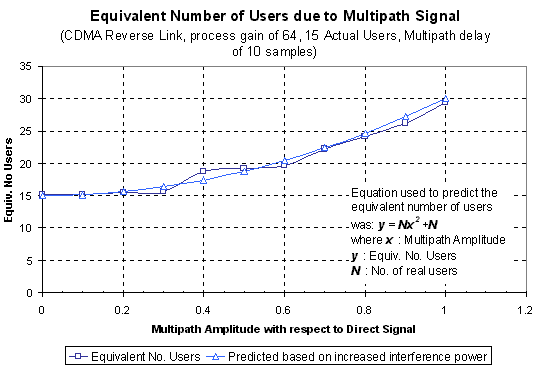Figure 36 Interference increase seen by the receiver due to multipath delay spread.  (x is the multipath amplitude, thus x2 is the multipath power)

### 3.2.3 Peak Power Clipping

The distortion tolerance of any transmission technique is very important, as it determines what type of power amplifier can be used, and how much dynamic range overhead is required. If a transmission technique is tolerant to peak power clipping, then it allows the signal to be clipped. This clipping of the signal reduces the peak to RMS signal power ratio thus allowing the signal power to be increased for the same sized transmitter. Figure 37 shows the effect of peak power clipping on both the reverse and forward links for CDMA.Figure 37 Effect of peak power clipping on the BER for the forward and reverse links of CDMA.

For the reverse link the BER starts high initially due to inter-user interference. The peak power clipping of the signal has little effect on the reverse link because the extra noise due to the distortion is not very high compared with the inter-user interference, plus any added noise is reduced by the process gain of the system. Peak power clipping for the reverse link is also likely to be small, as clipping would only ever occur due to distortion in the base station receiver, as this is the only point where all the signals are combined. A well-designed receiver is unlikely to cause significant clipping of the signal and thus the result shown in Figure 37 is not very important.

(Addendum 10/2001: CDMA mobile phones require that the CDMA signal is band-pass filtered before being transmitted. This is to prevent interference between neighbouring channels. This band pass filtering changes the transmitted signal from a simple binary phase transmission (i.e. constant amplitude) to one that varies continuously in amplitude. This variable amplitude requires linear amplification, and is thus affected by distortion. This means that some distortion is likely to result in each of the mobiles, as well as the base station).

The forward link result is more important as significant clipping of the transmitted signal could occur at the base station transmitter. The result for the forward link is completely different to the reverse link. The peak power clipping tolerance of the forward link is very similar to the result obtained for OFDM (see Figure 18 in section 2.2.2). The BER is low for a peak power clipping of less then 10dB, above which the orthogonal nature of the Walsh codes used begins to collapse.

### 3.2.4 Channel Noise

The noise performance of the CDMA reverse link is shown in Figure 38. This shows that the BER rises as the SNR of the channel worsen. Due to the high level of inter-user interference the addition of channel noise leads to only a gradual rise in the BER. The BER of each of the lines (10 user, 20 users and 30 users) approaches approximately the same BER at a SNR of 0 dB. At 0dB the effect noise of the channel is the same as adding an additional 64 users to the cell, thus the difference between 10, 20 and 30 users becomes insignificant. The BER is very bad for more than 10 users regardless of the channel SNR, thus making 20 or 30 users unusable. However, for 10 users the BER becomes greater the 0.01 at approximately a SNR of 14 dB, which is the maximum BER that can be normally tolerated for voice communications.Figure 38 BER verses the radio channel SNR for the reverse link of a CDMA system.

## 3.3 Mathematical Model for Reverse Link

### 3.3.1 Cell Capacity for a CDMA system

The capacity of a CDMA system is limited by the reverse link. The reverse link uses uncorrelated, non-orthogonal PN codes, which makes it limited by interference from other users. Each other user appears as noise as additional noise to the cell. If we initially assume a single cell then the noise in the system will be determined by the number of users in the cell. If we let the number of users be N, and the transmitted power from each user to be S, the received signal will consists of the received signal power for the desired user (S) and the interference from N-1 other users, thus the signal to noise ratio will be: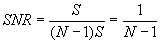Since the noise in the channel is reduced by the process gain during demodulation, the noise on each data bit seen after demodulation will be less. The process gain is the ratio of the total bandwidth (W) to the base band information bit rate (R). Thus the received energy per bit to noise ratio (Eb/No) isThe above equation does not take into account thermal noise. The thermal noise simply increased the effective amount of noise. Let the thermal noise be n. Thus, the Eb/No becomes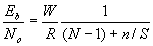In order to achieve an increased capacity, the interference from users needs to be reduced. This can be achieved by monitoring the voice activity so that the transmitter is switched off during periods of no voice activity. This reduces the effective interference level by the reduced duty cycle of the transmitted signal. The interference can also be reduced by using antenna sectorization. If for example the cell was sub divided using three antennas, each having a beam width of 120°, then the interference seen by each antenna is one third that of an omni-direction antenna. If we let d be the duty cycle of the voice activity, and G be the cell sectorization then equation becomes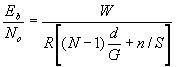Thus the capacity of a single cell CDMA system would be

Equation 2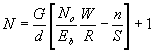Where:

G is the antenna sectorization,

d is the voice duty cycle,

Eb/No is the energy per bit to noise ratio,

W is the total transmission bandwidth,

R is the base band bit rate,

n/S is the ratio of received thermal noise to user signal power.

### 3.3.2 Capacity of a single CDMA cell

The cell capacity of a CDMA system is dependent on the bandwidth used the process gain and the allowable error rate. For this discussion we will consider the a system with the same bandwidth and user data rate the same as the OFDM example system in section 2.6.

The OFDM example used a bandwidth of 1.25MHz. The OFDM system could handle 64 users each at 39 kbps, or 128 users at 19.5 kbps depending on the spectrum allocation. For CDMA if we use a process gain of 64, this will give each user a data rate capacity of 19.5 kbps, making it comparable to an OFDM system with 128 users. Since the capacity of a CDMA system is dependent on the noise tolerance of data if we assume an Eb/No of  8dB this will give a BER of ~0.006 which is acceptable for voice communications (see Table 23 in Appendix III for more detail). For a the CDMA link that has no voice detection activity and no cell sectorization then cell capacity can be calculated using Equation 2 as follows:

G = 1, d = 1, Eb/No = 8 dB = 6.31, W = 1.25 MHz, R = 19.5 kHz and n/S = 0 (Assume no thermal noise)

From Equation 2: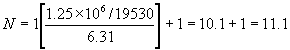This gives a spectral efficiency of only:This result is pretty poor as the cell capacity is more then 10 times lower then it was for OFDM. However the efficieny of CDMA can be improved by using voice detection to reduce the duty cycle of each user, and by using cell sectorization. Note however, that voice activity detection can only be used for voice communications and not for general data transfer. Thus all it is effectively doing is reducing the data throughput allowed for each user.

Appling both voice duty cycle detection and cell sectorization the effective capacity is increased. If we assume that the cell is split three ways then the ideal cell sectorization factor will be 3. However, sidelobes of the antennas used will always reduce this, there reducing the factor to about 2.55.

Using G = 2.55, d = 0.4 (i.e. 40%) the cell capacity becomes:The spectral efficiency is thus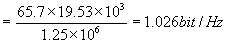This is still half the capacity of the OFDM system, and it comes at the cost of reduced total data throughput. Table 19 shows how the over capacity of the CDMA system changes depending on what BER is allowed. This is different to OFDM as the BER is ideally 0 even for 128 users as in the above example.

 Eb/No(dB) Expected Bit Error Rate (BER) Max. No. Users for single cell (no voice detection, no cell sectorization) Spectral Efficiency (bits/Hz) Max. No. Users for single cell (voice detection, cell sectorization) Spectral Efficiency (bits/Hz) 6 0.023007 17.1 0.267 103.6 1.62 8 0.006004 11.1 0.173 65.7 1.03 10 0.000783 7.4 0.116 41.8 0.65 12 3.43E-05 5.0 0.078 26.5 0.41

Table 19 Predicted cell capacity for a single CDMA cell with process gain of 64, depending on the tolerable Eb/No

### 3.3.3 Capacity of CDMA and OFDM with Multiple Cells

With any cellular system, interference from neighboring cells lowers the overall capacity of each cell. For conventional FDMA and TDMA systems, each cell must have a different operating frequency from its immediate neighboring cells. This is to reduce the amount of interference to an acceptable level. The frequencies are reused in a pattern, with the spacing between cells using the same frequency determined by the reuse factor. The required frequency reuse factor depends on the interference tolerance of the transmission system. Analog systems typically require a carrier to interference ratio (C/I) of greater then 18 dB , which requires reuse factor of 1/7 (see Figure 39 (b)). Most digital systems only require a C/I of 12 dB, allowing a reuse factor of 1/3-1/4 (see Figure 39 (a)). CDMA however, uses the same frequency in all cells, thus ideally allowing a reuse factor of 1 (see Figure 39).Figure 39 Frequency reuse patterns for (a) 3 frequencies (Digital systems), (b) 7 frequencies (Analog FDMA), (c) CDMA

In practice, the frequency reuse efficiency of CDMA is some what lower 1, as neighboring cells cause interference, thus reducing the user capacity of both systems. The frequency reuse factor for a CDMA system is about 0.65 . Figure 40 shows the interference from neighboring cells. Note that most of the neighboring interference is from the immediate neighbors of the cell.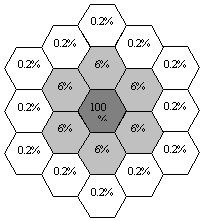Figure 40 Interference contributions from neighboring cells in a CDMA system (source ).

The cell capacity for a multi-cellular CDMA system equal to the single cell capacity reduced by the frequency reuse factor. Table 20 shows the effect of this on the CDMA capacity. The cell capacity for CDMA is very low if voice activity detection and cell sectorization is used. A straight CDMA system can only have somewhere between 5-11 users/cell/1.25 MHz. Using cell sectorization and voice activity detection allows the capacity to be increased by up to 6.4 time, allowing somewhere between 30-70 user/cell/1.25 MHz.

OFDM would require a frequency reuse pattern to be used in a multi-cellular environment to reduce the level of inter-cellular interference. The C/I required would need to be greater then ~12 dB (see Gaussian Noise Tolerance of OFDM, section 2.2.3). This could be done with a frequency reuse factor of ~3. This should easily be able to be achieved as cell sectorization could also be used to reduce the level of interference. This would result in the cell capacity for OFDM to be approximately equal to 128/3 = 42.7 users/cell/1.25 MHz in a multicellular environment. The matches the same user capacity as CDMA for an Eb/No of 8 dB and using cell sectorization and voice detection.

 Eb/No(dB) Expected Bit Error Rate (BER) Max. No. Users for single cell (no voice detection, no cell sectorization) Max. No. Users for single cell (voice detection, cell sectorization) 6 0.023007 11.1 67.3 8 0.006004 7.2 42.7 10 0.000783 4.8 27.2 12 3.43E-05 3.3 17.2

Table 20 Predicted cell capacity for a CDMA cell in a multi-cellular enviroment, for a process gain of 64.

Next Conclusion